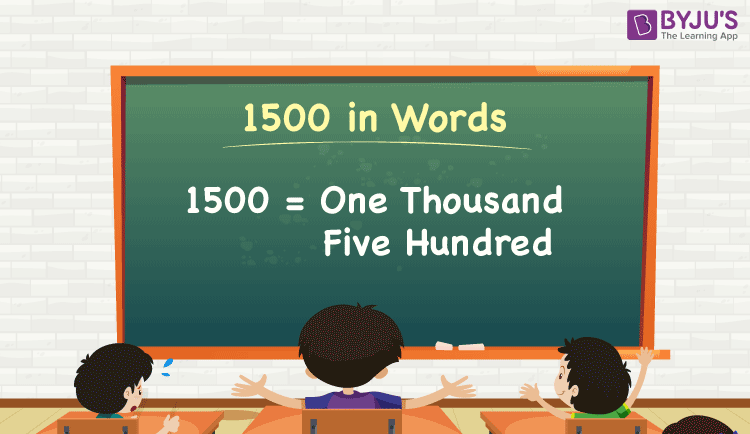# 1500 in Words

We can write 1500 in words as One thousand five hundred. Sometimes 1500 can also be written in words as Fifteen hundred. Suppose you have Rs. 1500 in your wallet, you can say that “I have One thousand five hundred rupees in my wallet”. It is possible to convert 1500 into words with the help of a place value chart. Also, we know that 1500 is a cardinal number since it represents a specific quantity.

 1500 in words: One thousand five hundred One thousand five hundred in numerical form: 1500

1500 in English Words

We generally write numbers in words using the English alphabet. Thus, we can read 1500 in English as “One thousand five hundred”.## How to Write 1500 in Words?

Let’s have a look at the conversion of 1500 into words using a place value chart. The number 1500 has four digits, so let’s make a chart that shows the place value up to 4 digits.

 Thousands Hundreds Tens Ones 1 5 0 0

Thus, we can write the expanded form as:

1 × Thousand + 5 × Hundred + 0 × Ten + 0 × One

= 1 × 1000 + 5 × 100 + 0 × 10 + 0 × 1

= 1000 + 500

= 1500

= One thousand five hundred

Therefore, 1500 in words is written as One Thousand Five Hundred.

Interesting way of writing 1500 in words:

1 = One

15 = Fifteen

150 = One hundred and fifty

1500 = One thousand five hundred (Or) Fifteen hundred

### Facts About the Number 1500

1500 is a natural number that precedes 1501 and succeeds 1499.

1500 in words – One thousand five hundred

Is 1500 an odd number? – No

Is 1500 an even number? – Yes

Is 1500 a perfect square number? – No

Is 1500 a perfect cube number? – No

Is 1500 a prime number? – No

Is 1500 a composite number? – Yes

### 1000 to 1500 in Words

1000 in words = One thousand

1100 in words = One thousand one hundred

1200 in words = One thousand two hundred

1300 in words = One thousand three hundred

1400 in words = One thousand four hundred

1500 in words = One thousand five hundred

## Frequently Asked Questions – FAQs on 1500 in Words

Q1

### How to Write the Number 1,500 in Words?

1,500 in the word is written as one thousand five hundred.
Q2

### State True or False. Is 1,500 Divisible by 5?

True.1,500 is divisible by 5.
Q3

### Is 1,500 a Perfect Cube Number?

No.1,500 is not a perfect cube.
Q4

### What is the Value of One Thousand Five Hundred Minus One Hundred and Thirty?

1,500 – 130 = 1,370, which is read as One Thousand Three Hundred Seventy.
Q5

### Find the Value of 338 + 1162. Write the Answer in Words.

338 + 1162 = 1500.Which is written as One Thousand Five Hundred.